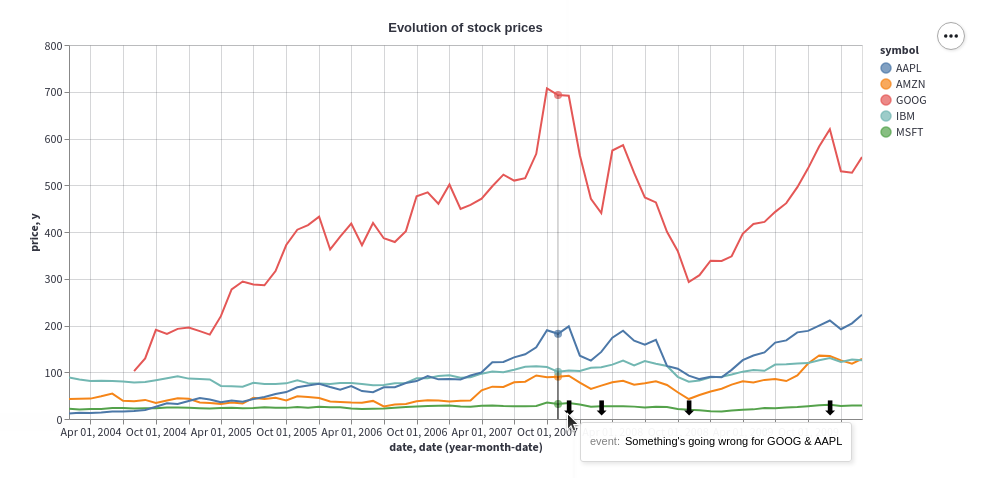Display a chart using the Altair library.
Function signature

st.altair_chart(altair_chart, use_container_width=False)

Parameters

altair_chart (altair.vegalite.v2.api.Chart)

The Altair chart object to display.

use_container_width (bool)

If True, set the chart width to the column width. This takes precedence over Altair's native width value.

#### Example

import pandas as pd
import numpy as np
import altair as alt

df = pd.DataFrame(
np.random.randn(200, 3),
columns=['a', 'b', 'c'])

c = alt.Chart(df).mark_circle().encode(
x='a', y='b', size='c', color='c', tooltip=['a', 'b', 'c'])

st.altair_chart(c, use_container_width=True)


Examples of Altair charts can be found at https://altair-viz.github.io/gallery/.

(view standalone Streamlit app)

Altair also allows you to annotate your charts with text, images, and emojis. You can do this by creating layered charts, which let you overlay two different charts on top of each other. The idea is to use the first chart to show the data, and the second chart to show the annotations. The second chart can then be overlaid on top of the first chart using the + operator to create a layered chart.

Let's walk through an example of annotating a time-series chart with text and an emoji.

#### Step 1: Create the base chart

In this example, we create a time-series chart to track the evolution of stock prices. The chart is interactive and contains a multi-line tooltip. Click here to learn more about multi-line tooltips in Altair.

First, we import the required libraries and load the example stocks dataset using the vega_datasets package:

import altair as alt
import pandas as pd
import streamlit as st
from vega_datasets import data

# We use @st.experimental_memo to keep the dataset in cache
@st.experimental_memo
def get_data():
source = data.stocks()
source = source[source.date.gt("2004-01-01")]
return source

source = get_data()


Next, we define a function get_chart() to create the interactive time-series chart of the stock prices with a multi-line tooltip. The x-axis represents the date, and the y-axis represents the stock price.

We then invoke get_chart() that takes the stock prices dataframe as an input and returns a chart object. This is going to be our base chart on which we will overlay the annotations in Step 2.

# Define the base time-series chart.
def get_chart(data):
hover = alt.selection_single(
fields=["date"],
nearest=True,
on="mouseover",
empty="none",
)

lines = (
alt.Chart(data, title="Evolution of stock prices")
.mark_line()
.encode(
x="date",
y="price",
color="symbol",
)
)

# Draw points on the line, and highlight based on selection
points = lines.transform_filter(hover).mark_circle(size=65)

# Draw a rule at the location of the selection
tooltips = (
alt.Chart(data)
.mark_rule()
.encode(
x="yearmonthdate(date)",
y="price",
opacity=alt.condition(hover, alt.value(0.3), alt.value(0)),
tooltip=[
alt.Tooltip("date", title="Date"),
alt.Tooltip("price", title="Price (USD)"),
],
)
)
return (lines + points + tooltips).interactive()

chart = get_chart(source)


#### Step 2: Annotate the chart

Now that we have our first chart that shows the data, we can annotate it with text and an emoji. Let's overlay the ⬇ emoji on top of the time-series chart at specifc points of interest. We want users to hover over the ⬇ emoji to see the associated annotation text.

For simplicity, let's annotate four specific dates and set the height of the annotations at constant value of 10.

star

#### Tip

You can vary the horizontal and vertical postions of the annotations by replacing the hard-coded values with the output of Streamlit widgets! Click here to jump to a live example below, and develop an intuition for the ideal horizontal and vertical positions of the annotations by playing with Streamlit widgets.

To do so, we create a dataframe annotations_df containing the dates, annotation text, and the height of the annotations:

# Add annotations
ANNOTATIONS = [
("Mar 01, 2008", "Pretty good day for GOOG"),
("Dec 01, 2007", "Something's going wrong for GOOG & AAPL"),
("Nov 01, 2008", "Market starts again thanks to..."),
("Dec 01, 2009", "Small crash for GOOG after..."),
]
annotations_df = pd.DataFrame(ANNOTATIONS, columns=["date", "event"])
annotations_df.date = pd.to_datetime(annotations_df.date)
annotations_df["y"] = 10


Using this dataframe, we create a scatter plot with the x-axis representing the date, and the y-axis representing the height of the annotation. The data point at the specific date and height is represented by the ⬇ emoji, using Altair's mark_text() mark.

The annotation text is displayed as a tooltip when users hover over the ⬇ emoji. This is achieved using Altair's encode() method to map the event column containing the annotation text to the visual attribute ⬇ of the plot.

annotation_layer = (
alt.Chart(annotations_df)
.mark_text(size=20, text="⬇", dx=-8, dy=-10, align="left")
.encode(
x="date:T",
y=alt.Y("y:Q"),
tooltip=["event"],
)
.interactive()
)


Finally, we overlay the annotations on top of the base chart using the + operator to create the final layered chart! 🎈

st.altair_chart(
(chart + annotation_layer).interactive(),
use_container_width=True
)To use images instead of emojis, replace the line containing .mark_text() with .mark_image(), and replace image_url below with the URL of the image:

.mark_image(
width=12,
height=12,
url="image_url",
)


#### Interactive example

Now that you've learned how to annotate charts, the sky's the limit! We've extended the above example to let you interactively paste your favorite emoji and set its position on the chart with Streamlit widgets. 👇

(view standalone Streamlit app)